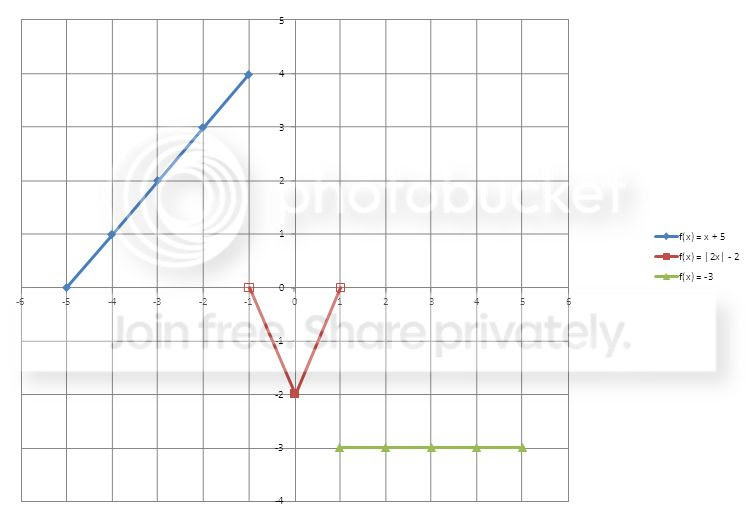f(x)= { x+5 |2x| - 2 -3 -5 ≤ x ≤ -1 -1 < x 11 ≤ x ≤ 5

Evaluate each x values for each function.

 If the x value is from -5 to -1, f(x) = x + 5 Substituted function f(-5) = -5 + 5 = 0 f(-4) = -4 + 5 = 1 f(-3) = -3 + 5 = 2 f(-2) = -2 + 5 = 3 f(-1) = -1 + 5 = 4 Point (-5, -0) (-4, 1) (-3, 2) (-2, 3) (-1, 4) If the x value is between -1 to 1, f(x) = |2x| - 2 f(-1) = |2(-1)| - 2 = 0 f(0) = |2(0)| - 2 = -2 f(1) = |2(1)| - 2 = 0 (-1, 0) (0, -2) (1, 0) If the x value is from 1 to 5, f(x) = -3 f(1) = -3 f(2) = -3 f(3) = -3 f(4) = -3 f(5) = -3 (1, -3) (2, -3) (3, -3) (4, -3) (5, -3)
Here is the graph:Click to enlarge

Notice that points (-1,0) and (1,0) are hollow points. It means these points are not included in the range.